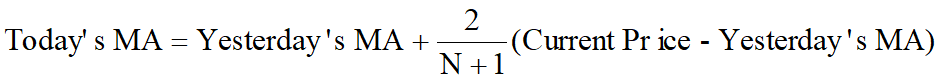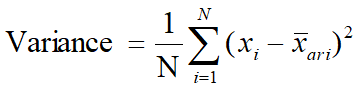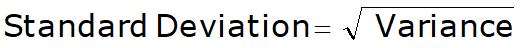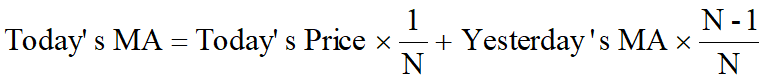﻿ Glossary of Terms

# Glossary of Terms

This is not a complete glossary because some knowledge of trading concepts is assumed. This Glossary contains the most important definitions used in Trading Blox.

Average True Range (ATR)

The Average True Range is a measure of volatility which indicates the average range of price movement in a single day. This measure was developed by J. Welles Wilder and introduced in his book, New Concepts in Technical Trading Systems (1978).

The average of the True Range where True Range is the range including opening gaps, or the greatest of:

The current high minus the current low

The current high minus the previous close

The previous close minus the current low

The last two entries account for price gaps where the entire day's prices do not overlap the previous day's close.

Trading Blox allows you to set the number of days to use when creating an Average True Range Indicator.  In the Trading Blox supplied systems and optional blox can use either an Exponential Moving Average method or a Simple Average method to calculate this indicator.  In the Builder version of Trading Blox other averaging methods can be scripted and used as well. For our purposes here it is important to note that the Exponential Moving Average is a different formula than the Wilder Moving Average Formula used by Wells Wilder in his book "New Concepts in Technical Trading Systems." As an example, the 20 day Wilder ATR is the equivalent to a 39 day Exponential ATR.

Exponential MA Days = (Wilder MA Days X 2) - 1

Autocorrelation

A term referring to the correlation of a time series with a lagged version of itself. Sometimes referred to as serial correlation or lagged correlation.

Breakout

A chart pattern used to indicate a rise in a stock's price above its resistance level or drop below its support level. It is typically measured in terms of days. An N-Day breakout is a breakout of the highest high of the last N Days or the lowest low of the last N Days, where N is the number of days for the breakout. Thus a 20-Day breakout of the highs would occur when the price exceeded the highest high of the last 20 days.

Breakouts are used in trend-following systems to indicate a potential start of a trend.

Bollinger Band

A Bollinger Band is a pricing channel which consists of a top and bottom price which are defined in terms of a Simple Moving Average and the price a fixed number of Standard Deviations above that moving average, and below that moving average.

Closed Equity

Closed Equity is a measure of equity that take the starting balance and adjusts for the profits of closed out trades only. It does not take into account the value of open positions.

Confidence Level

A Confidence Level is used during Monte Carlo Simulations to indicate that a certain percentage of the simulated equity curves demonstrated results for a particular measure that were greater than the confidence level. For example, a 95% Confidence Level MAR Ratio of 0.8 indicates that 95% of the simulation iterations showed a MAR Ratio of 0.8 or better.

Core Equity

Core Equity is a measure of equity that includes Closed Equity plus that portion of Open Equity that would be realized if all the current positions were exited at their current stop values (i.e. Locked-In Profits).

Core Equity = Closed Equity + Locked-In Profits

# Expectation:

( TotalWinPercent - TotalLossPercent ) / TotalRiskPercent

# Total Risk Percent = sum of ( percent of trade entry equity risked ) of all trades

Exponential Moving Average

Similar to a simple moving average (SMA), except that more weight is given to the latest data. Also known as an exponentially weighted moving average, this type of moving average reacts more quickly to recent price changes than a Simple Moving Average. Our Exponential Moving Averages start with a simple moving average of the number of days in the moving average, and then use the Exponential formula thereafter.

An Exponential Moving Average is computed according to the following formula:

#Exponential MA Days = (Wilder MA Days X 2) - 1

Forex Carry

An adjustment for the costs of borrowing and lending the two currencies involved in a Forex transaction.

Locked-In Profits

That portion of Open Equity that would be realized if all the current positions were exited at their current stop values.

MAR Ratio        This statistic, developed by the Managed Accounts Report newsletter, is a simple ratio that divides the CAGR (Compound annual growth rate) by the test periods biggest draw down percentage.  For example, when a test shows a CAGR = 19.08% and it has it Largest Drawdown = 29.1% then results are: (19.08 / 29.1 ) = 0.655670

Moving Average

An average measure of prices that is computed using a fixed number of days. For example, a 20 day moving average is computed using the last 20 days. There are two common types of moving averages: Simple Moving Average, and Exponential Moving Average.

N

A measure of volatility, N is a concept used by the Turtles (student's of Richard Dennis and Bill Eckhardt) to represent the underlying volatility of a particular market.

N is simply a 20-day Average True Range. Conceptually, N represents the average range in price movement that a particular market makes in a single day, accounting for opening gaps. N was measured in the same points as the underlying contract.

See the Original Turtle Rules Document for more info on N as used by the Turtles.

Open Equity

A measure of the value of positions that have been entered but not closed out. In Trading Blox, Open Equity is the current value of each position measured at the close of the previous day.

For a given long position:

Position Open Equity = (Current Price - Entry Price) X Contracts or Shares X Dollars per Point

For a given short position:

Position Open Equity = (Entry Price - Current Price) X Contracts or Shares X Dollars per Point

Percent Profit Factor

A measure of the relative profitability of a system. Like Profit Factor below except it is calculated using:

Percent Profit Factor = Average Win Percent / Average Loss Percent

Profit Factor

A measure of the relative profitability of a system. Profit Factor is calculated using:

Profit Factor = Total Winning Trade Profits / Total Losing Trade Losses

R-Multiple™

The concept of an R-Multiple™ was pioneered in 1993 by private trader Chuck Branscomb. R-Multiple™ is short for "Risk Multiple".

An R-Multiple™ is simply the profit or loss for a given trade divided by the entry risk. The entry risk is defined as the difference between the entry price and the stop price as the time of the entry.

'  R-Multiple Calculation Example
'  Profit / ( ( entry fill price - entry protective stop ) * quantity )
2243.68 / ( ( 8.27 - 8.25 ) * 1516 ) = 74

To learn about the origin of R-Multiple™s, see the forum topic Improve Risk/ Reward by using Multiple Timeframes on the Trader's Roundtable forum.

R Squared

A statistical measure of how close an equity curve is to a straight line plotted on a logarithmic graph. A value of 0 indicates a jagged line and a value of 1.0 represents a straight line. A fixed percentage investment that compounded and paid daily would have a straight line equity curve and an R Squared value of 1.0.

Set File

A Set file is an ASCII Text file which defines a portfolio which will appear in the Portfolio manager section of each system. See: Portfolio Editor for more information.

Simple Moving Average

A simple, or arithmetic moving average is calculated by adding the closing price of the security or futures market for a number of time periods, then dividing this total by the number of time periods.  In other words, it's the average stock price over a period of time. Unlike the Exponential Moving Average, this type of moving average gives equal weight to each daily price.

Standard Deviation

In trading, a Standard Deviation of price data is often used as a measure of volatility. In this context it is usually measured over a fixed number of days rather than over the entire data set.

More generally, the standard deviation (SD) of a given data set is derived from its variance, i.e. the arithmetic mean of all the squared distances between the data values and their arithmetic mean:

#The standard deviation is defined as the square root of the variance:

#Total Equity

Total Equity is a measure of equity that takes into account all closed out trades and open position equity (or Open Equity).

Total Equity = Closed Equity + Open Equity

Wilder Moving Average Formula

Welles Wilder's indicators, including Average True Range do not use the standard exponential moving average formula. The Wilder formula for computing an N-Day Exponential Moving Average is:

#You can determine the equivalent non-Wilder using the following formula:

Exponential MA Days = (Wilder MA Days X 2) - 1

 Edit Time: 9/12/2020 9:50:00 AM Topic ID#: 150

Created with Help & Manual 7 and styled with Premium Pack Version 2.80 © by EC Software